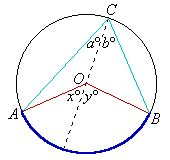kwizNET Subscribers, please login to turn off the Ads!
 Email us to get an instant 20% discount on highly effective K-12 Math & English kwizNET Programs!

#### Online Quiz (WorksheetABCD)

Questions Per Quiz = 2 4 6 8 10

### High School Mathematics - 28.5 Angle at Centre Theorem

 : Angle subtended by an arc at the centre is twice the angle subtended by the angle at the circumference.Given: ACB is the major arc of the circle. Angles AOB and ACB stand on the same arc. To prove: Angle AOB = 2 Angle ACB Proof: From triangle AOC, x = a+a (Exterior angle of a triangle) Hence x = 2a From triangle OBC, y = b+b Hence y = 2b Angle AOB = x + y Hence angAOB = 2(a+b) Hence Angle AOB = 2 Angle ACB Example: Find angle a.Angle at circumference = 1/2 angle at centre. Angle a = 70o Answer: 70o Directions: Solve the following
 Q 1: Answer: Q 2: Find angle ACB.54o90o27o Q 3: If the sum of the angles at the circumference and the centre is 120 degrees. Find the angle at centre.70o80o40o Q 4: Consider triangle ABC in a circle, if one angle at the centre is 200 degrees, what is the other angle at the centre?100o160o120o Q 5: Answer: Q 6: If one angle at the circumference of a circle is 55 degrees , find the angle at centre.100o55o110o Q 7: Answer: Q 8: Consider triangle ABC inscribed in a circle, if ang ABC is 50 degrees and is in the major arc, what is the angle at the centre.50o100o75o Question 9: This question is available to subscribers only! Question 10: This question is available to subscribers only!

#### Subscription to kwizNET Learning System offers the following benefits:

• Unrestricted access to grade appropriate lessons, quizzes, & printable worksheets
• Instant scoring of online quizzes
• Progress tracking and award certificates to keep your student motivated
• Unlimited practice with auto-generated 'WIZ MATH' quizzes
• Child-friendly website with no advertisements
• Choice of Math, English, Science, & Social Studies Curriculums
• Excellent value for K-12 and ACT, SAT, & TOEFL Test Preparation
• Get discount offers by sending an email to discounts@kwiznet.com

 Quiz Timer